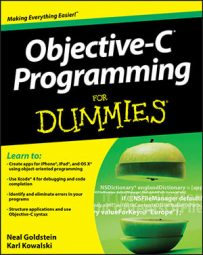##### Objective-C Programming For DummiesObjective-C operators, like those in other programming languages, let you perform operations on variables (hence the name). Objective-C provides many operators, and keeping track of all of them can be difficult as you program your iOS or Mac OS X apps. Use the following tables to jog your memory as to which operator accomplishes what task.

Arithmetic Operators
Operator What It Does
- Subtraction
* Multiplication
/ Division
% Modulo
Relational and Equality Operators
Operator What It Does
== Equal to
!= Not equal to
> Greater than
< Less than
>= Greater than or equal to
<= Less than or equal to
Logical Operators
Operator What It Does
! NOT
&& Logical AND
|| Logical OR
Compound Assignment Operators
Operator What It Does
-= Subtraction
*= Multiplication
/= Division
%= Modulo
&= Bitwise AND
|= Bitwise Inclusive OR
^= Exclusive OR
<<= Shift Left
>>= Shift Right
Increment and Decrement Operators
Operator What It Does
++ Increment
-- Decrement
Bitwise Operators
Operator What It Does
& Bitwise AND
| Bitwise Inclusive OR
^ Exclusive OR
~ Unary complement (bit inversion)
<< Shift Left
>> Shift Right
Other Operators
Operator What It Does
() Cast
, Comma
Sizeof() Size of
? : Conditional